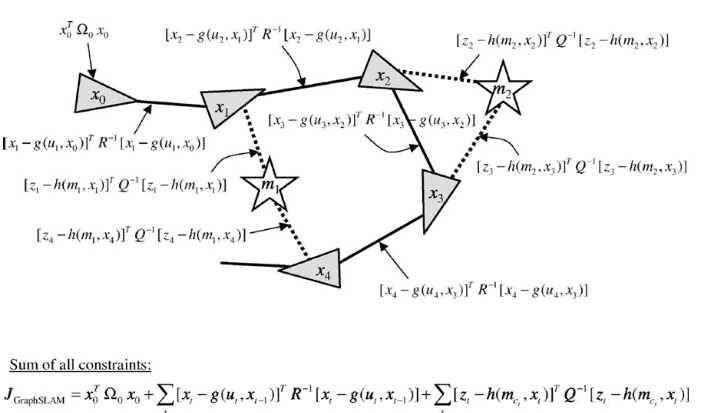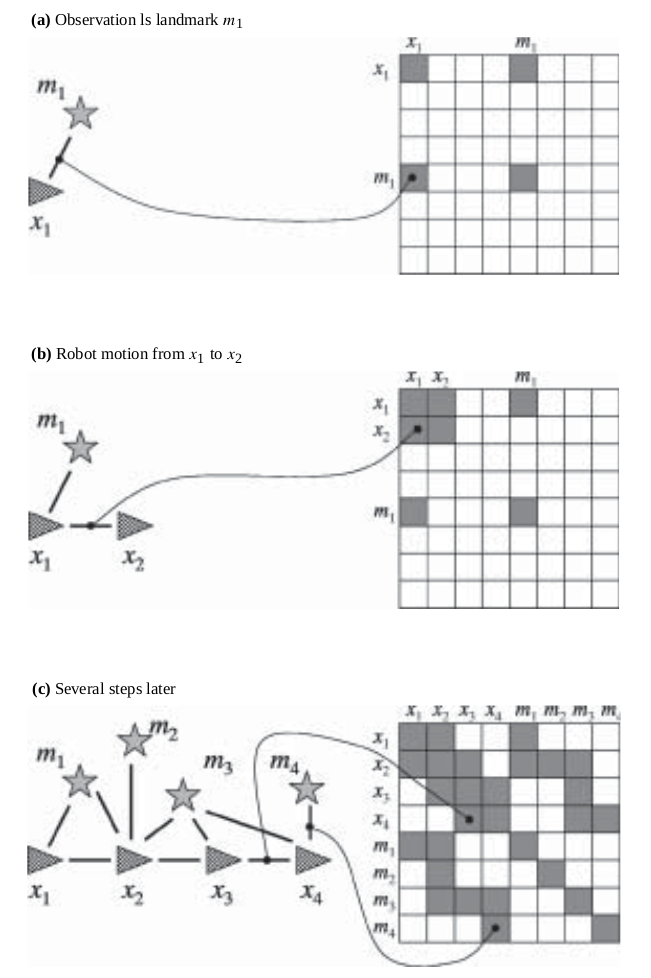0%

### 从 SLAM 问题构建图

#### 离线 SLAM 问题介绍

• $x_{1:t}$ 表示机器人从 t = 1 到当前 t 时刻的位姿集合
• $m$ 作为环境（地图），有一系列特征点 $m_j$ 组成
• 同时机器人在某一个时刻可以对环境进行感知，通过观察多个特征来获得多个观测值 $z_t^i$，i 表示在该时间内机器人的第 i 个观测值。一般来说会假定 $z_T^i$ 是一个范围观测量。

GraphSLAM 可以适用于以上两种观测函数（将地图特征点作为观测对象和将机器人位姿作为观测对象）。

$\delta_t \sim \mathcal{N}(0, R_t)$ 作为控制指令的噪声，这个函数也可以作为机器人的运动学模型。同样，用概率的方式，转变函数也可以写成以下形式：

#### GraphSLAM 的基本思想• $x_0, x_1, …, x_4$ 四个时刻的机器人的位姿
• $m_1, m_2$ 两个地图特征点

• 两个相邻位姿之间的相对运动运动构成的边（实线）
• 某一位姿和特征点的观测误差构成的边（虚线）

#### 图的构建

• 机器人位姿 $x_{1:t}$
• 地图中的特征点 $m_{j}$

• 一次运动产生两个相邻机器人位姿节点之间的一条边
• 一次观测产生一个机器人位姿节点和特征点的一条边• 首先考虑一次测量值 $z_t^i$，这次测量提供了在机器人 $t$ 时刻的位姿 $x_t$ 和地图中 $j = c_t^i$ 特征点之间的信息。在 GraphSLAM 中，这个信息用一个在 $x_t$ 和 $m_j$ 之间的约束来表示。我们可以把这条边想象成弹簧质量系统里面的一个“弹簧”。约束可以表示成以下形式，其中 $h$ 表示观测模型函数， $Q_t$ 表示测量噪声的方差矩阵：

• 对于两个相邻的位姿 $x_{t-1}$ 和 ${x_t}$ 之间会有表示控制量的信息边引入
• 对于一个位姿 $x_t$ 和在该位姿观测到的特征点 $m_j$ 也会有一条边引入